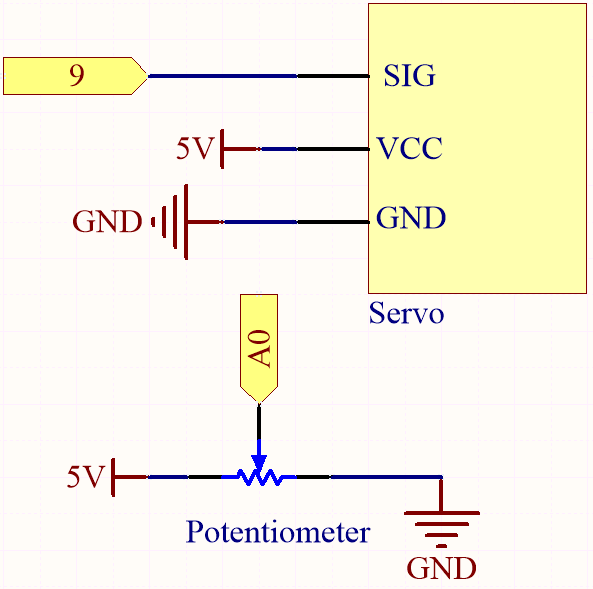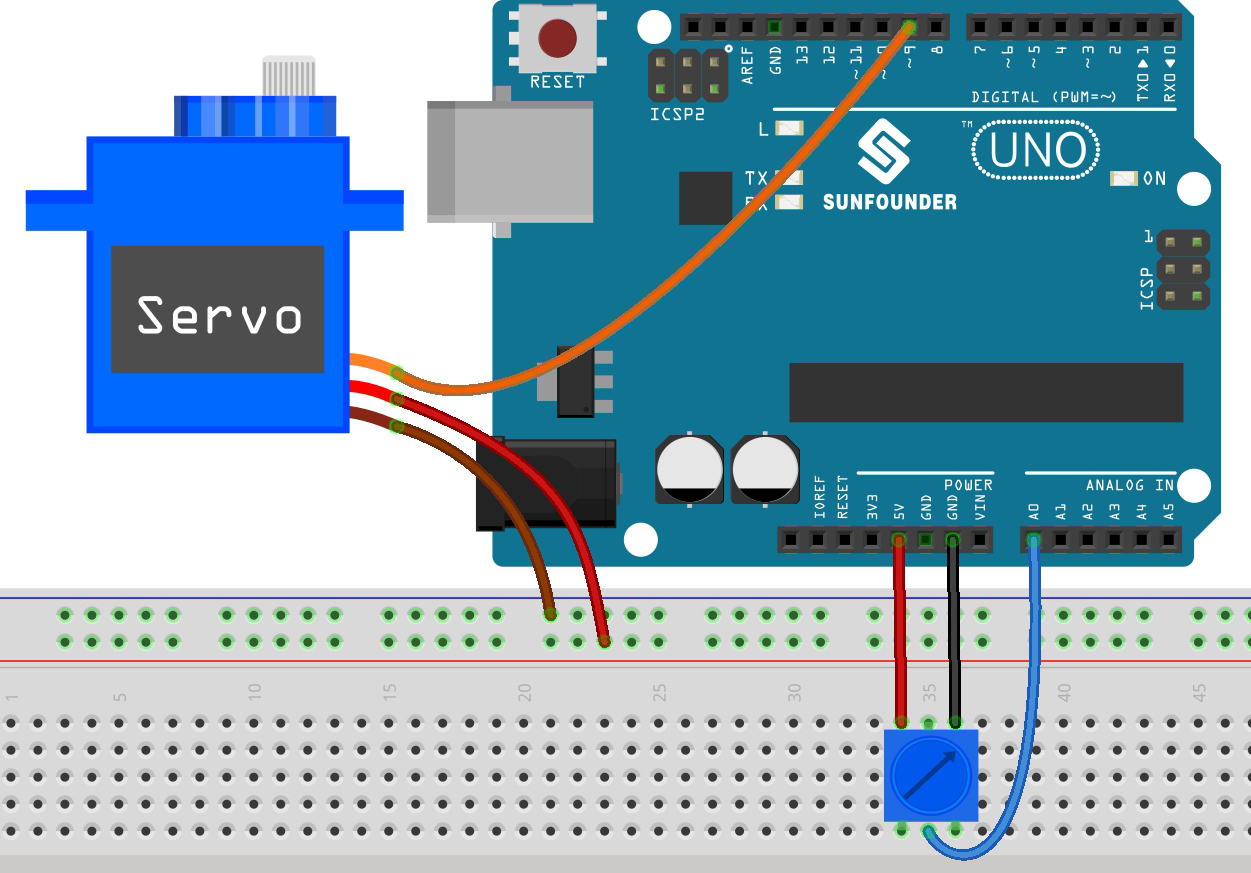# 5.6 Map¶

If you observe carefully, you will notice that many values have different ranges in programming. For example, the range of values for analog inputs is (0~1023). The value range for the analog output is (0~255). The output angle of the servo is (0~180).

This means that if we want to use the potentiometer to control the brightness of the LED or the angle of the servo, we need to go through a mapping operation.

Now let’s see how to achieve it.

Required Components

In this project, we need the following components.

Name

ITEMS IN THIS KIT

3 in 1 Starter Kit

380+

COMPONENT INTRODUCTION

SunFounder R3 Board

Jumper Wires

Servo

Potentiometer

SchematicWiringCode

Note

• Open the `5.6.map.ino` file under the path of `3in1-kit\basic_project\5.6.map`.

• Or copy this code into Arduino IDE.

• Or upload the code through the Arduino Web Editor.

After the code is uploaded successfully, you can rotate the potentiometer back and forth, and the output shaft of the servo will rotate back and forth.

How it works?

`map(value, fromLow, fromHigh, toLow, toHigh)`: Map a number from one range to another. That is, a fromLow value is mapped to toLow, and a fromHigh value is mapped to toHigh.

Syntax

map(value, fromLow, fromHigh, toLow, toHigh)

Parameters
• `value`: the number to map.

• `fromLow`: the lower bound of the value’s current range.

• `fromHigh`: the upper bound of the value’s curr+ent range.

• `toLow`: the lower bound of the value’s target range.

• `toHigh`: the upper bound of the value’s target range.

If the potentiometer controls the LED, you can also use the map to complete the task.

```int x = analogRead(knob);
int y = map(x,0,1023,0,255);
analogWrite(led,y);
```

Notes and Warnings

• The “lower bound” of both ranges may be larger or smaller than the “upper bound”, which means that the `map()` function can be used to reverse a range of numbers.

```y = map(x,0,180,180,0);
```
• Mapping also works well for negative numbers.

```y = map(x,0,1023,-90,90);
```
• The mapping uses integers, and the decimal places of floats are discarded.